# 05 - C Operators

This chapter will introduce operators in C language. Operator is a symbol which specifies the operation to be performed. We can say, operators carry out arithmetic and logical operations.  Operands are the values which can be operated by operators. The operators on C can be broadly classified on the following two categories:

• Number of operands
• Type of operation be performed

## 5.1 Operators classified on the basis of number of operands

C operators operate on a single operand or more than one operands. They are used to perform basic arithmetic operations, comparison operations, manipulation of bits, etc. These operators are of following types:

1.Unary Operator

Example: -21 , +45, etc.

2.Binary Operator: Binary operator works on two operands to produce result. Type of expression formed by these operators have the operator in between two operands.

Example: 21 + 45, a*b, etc.

1. Ternary Operator: Ternary operator is also known as conditional operator. This operator involves three operands to produce result.

Example: a?b:c

## 5.2 Operators classified on the basis of type of operation

Operators are categorized depending on the types of operation performed and they are as follows:

• Arithmetic operators
• Assignment operators
• Increment or decrement operator
• Relational operators
• Logical operators
• Conditional operators
• Bitwise operators
• Special operators
• Comma operator
• sizeof operator

### 5.2.1 Arithmetic operators

These operators are used to perform arithmetic expressions for example, addition, subtraction, multiplication, division, etc. Exponentiation operation is performed by pow() but it is a library function. Modulus operator is used to find the remainder after an integer division.Figure - C arithmetic binary operators

Addition operation: 4 + 2 = 6

Subtraction operation: 4 – 2 = 2

Multiplication operation: 4 * 2 = 8

Division operation: 4 / 2 = 2

Modulus operation: 4 % 2 = 0

#### 5.2.1.1 Arithmetic Expression

Arithmetic expression can be rightly defined as an expression which consists of constants and variables which performs meaningful calculation according to the arithmetic operators. These arithmetic operators which are involved in arithmetic expression are known as Operands.

Evaluation of arithmetic expression is governed by certain rules. These rules are known as Precedence Rules or Precedence of operators. In other words, there is a preference maintained for arithmetic operators while evaluating the expression. Let us use a small term to make it easy to remember: BODMAS. Let us decipher this small magical code.

• B-Brackets will always have first preference
• O-Of operator will have second preference
• D-Division operator is going to get the third preference
• M-Multiplication operator is going to get the fourth preference
• A-Addition operator is going to get the fifth preference
• S-Subtraction operator is going to get last preference

Let us consider an example: Expression: 6 * (5 – 3) + 2

Step 1: 6 * (2) + 2

Step 2: 12 + 2

Step 3: 14

Solution: 14

Now it is not mandatory that you are going to get an easy to evaluate expression where you can easily apply the BODMAS rule. What if an expression consists two or more operators with same preference priority. In C language, each and every operator has an associated proprity value and expressions are evaluated according to them. We have solution for this problem.

• Left-to-right associative: The operators with same priority in an expression are evaluated from left to right. These operators are also called Left to Right associative operators and the process of evaluating is known as Left associative. It is denoted by L->R.
• Example: 2 + 5 + 7 – 2
• Step 1: 2 + 5 + 7 – 2
• Step 2: 7 + 7 – 2
• Step 3: 14 – 2
• Solution: 12

Precedence of Operators: As we already mentioned, operators are assigned priority. The operations which are performed on the basis of precedence of operators are called Hierarchy of operations. Following list will help you in understanding this concept better: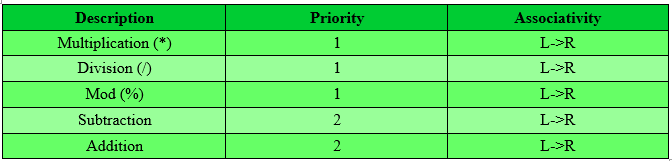Table: C Description of Operator precedence

#### 5.2.1. 2 Types of Arithmetic Expressions

Based on types of operands in an expression we broadly categorize them into 3 categories and they are:

• Integer Expression
• Floating point Expression
• Mixed mode Expression '

a) Integer Expression: An expression which contains integer type constants and variables hence the resulting expression will be of integer value and such an expression is known as Integer expression.

Let us consider some examples:

Expression: 17 / 3 = 5

Explanation: Let us consider the above expression, here both the operands are of type int so the result was formatted to 5 although the actual result is 5.667. In the result, value after decimal point is discarded.

Expression: 15 / 3 = 5

Explanation: All of us know the fact that when number 3 is multiplied 5 times, the result is 15 so when 15 divided by 3 is equal to 5.

b) Floating point Expression: An expression whose constituent variables and constants are of type floating point and double are known as Floating point Expression. Let us consider some examples:

Expression: 15.0 / 3.0 = 5.0

Explanation: Since both the operands are of type float so the result obtained is of type float.

Expression: 17.0 / 3.0 = 5.666

Explanation: When number 17 is divided by 3, the remainder is 2.

3 ) 17 ( 5.666

15

20

18

20

18

20

18

2

c) Mixed Mode Expression: These expressions consists of more than one type of operands. In other words, the constants and variables are of different data types like int, float, double etc in same expression. While expression is evaluated it should be of same data type so conversion of data takes place from one data type to another. This is known as implicit type conversion. Lower data type is converted to higher data type usually. Let us consider some examples:

Expression: 17.0 / 3 = 5.666

Explanation: In this expression, 3 is converted into float type then expression is evaluated. Then the result obtained is of type float.

Expression: 15. / 3 = 5.0

Explanation: In this expression, 3 is converted into float type then expression is evaluated. Then the result obtained is of type float.

### 5.2.2 Increment Operator

++ is known as Increment operator and it is an unary operator. This operator increments the  operand value by one. This operator is again of two types and they are as follows:

1.Pre increment operator: In this case, operator ++ is placed before the operand and hence value of operand is incremented before the value is used.

Example: ++ x

2.Post increment operator: In this case, operator ++ is placed after the operand and hence value of operand is used first and then increment the value by 1.

Example: x++

Now you must be thinking what is the difference if both of them are going to increment the value by 1. Difference comes into picture when these operators are a part of expression otherwise they are going to output same value.

### 5.2.3 Decrement Operator

Decrement operator is an unary operator. In contrast to increment operator, decrement operator decreases the value by 1. This operator is again of two types and they are as follows:

1. Pre decrement operator: In this case, operator -- is placed before the operand and hence value of operand is decremented before the value is used.

Example: -- x

2. Post decrement operator: In this case, operator -- is placed after the operand and hence value of operand is used first and then decrements the value by 1.

Example: x--

Now you must be thinking what is the difference if both of them are going to decrease the value by 1. Difference comes into picture when these operators are a part of expression otherwise they are going to output same value.

### 5.2.4 Assignment Operator

Assignment operator is used to store value in memory. In the last chapter, we studied about variables. Variable name is identifies the memory location and this assignment operator is used to copy the data or output of an expression into respective memory location. This process of copying data into memory location is called Assigning.

Format of Expression: variable_name = expression;

Let us consider some examples so that it becomes more clear to you.

Example: int a = b;

Explanation: In the above example, value of b is copied / stored into memory location identified by variable a which in turn is of type integer.

Example:       int length=5;

int area = length * breadth;

Explanation: In the above example, value 5 I stored into variable length which is of type integer. Similarly, integer variable breadth is assigned value 5. in the third line, value obtained after multiplying length and breadth is stored into the variable identified by variable area which is of type integer. So the value of area will be 25.

Short hand assignment operators: Short hand assignment operators are like short hand notations. Say, our friends call us by short names similarly this language has some short hand assignment operators. These operators are listed in the following tableTable - C Short hand assignment operators

### 5.2.5 Relational operators

Relational operators are used for comparison. As the name suggests, they are used to determine the relationship which exists between two operands. By operands, we mean constants, variables or expressions. Result of operational operators can be true or false. True is indicated by value 1 and false by 0 respectively. Following table enlists the relational operatorsTable  - C Relational operators

### 5.2.6 Logical operators

Logical operators are used to evaluate logical expressions. Logical operations are AND, OR NOT. The result of logical operators is 1 or 0. Logical operators are used to compare or combine two or more relational expressions. Following table lists the logical operators used in C languageTable - C Logical operators

Many of you might not be aware of the logic gates or logical operations we are talking about. So a preliminary explanation of all three logical operations are listed below:

• AND operation: This performs multiplication operation on logical . Result of ANDing is true only if both the operands are true, otherwise the value is false. True is indicated by 1 and false by 0. Following table shows AND operation: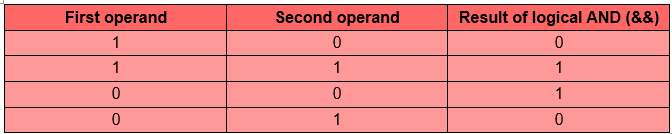Table - C Logical AND operation

• OR operation: This performs logical addition on operands. ORing outputs true if any of the logical operand is true. Result is false only if both the operands are false. True is indicated by 1 and false is indicated by 0. Following table shows OR operation: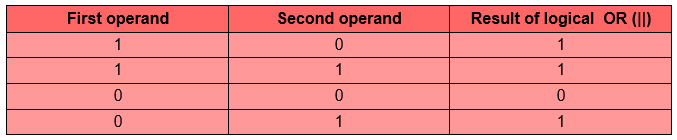Table - C Logical OR operation

• NOT operation: This is known as logical NOT. This operator logically complements or inverses the operand to which it is applied to. Result of logical NOT is true when operand is false and vice-verse. True is indicated by 1 and false is indicated by 0. Following table shows NOT operation:Table – C Logical NOT operation

### 5.2.7 Conditional operator

This operator is known as Ternary operator. This works on three operands. Conditional operators are ? :.

Format: expression1 ? first_value : second_value

Example: min = (a<b) ? a : b;

Explanation: min will be assigned the result of expression (a<b). If result is true then min will be assigned value a, otherwise b.

### 5.2.8 Bitwise operators

These are known as bit manipulation operators. With these opeartors we can manipulate constituent bits of a piece of data individually. These operators can only operate on integer and char input. They do not support float or double data types respectively. Data is stored I  memory as binary digits (0's and 1's).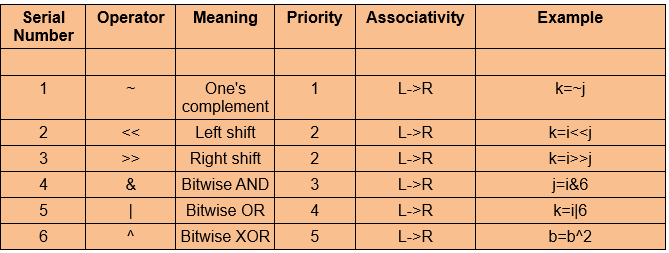Table - C Bitwise operators

Let us consider the examples for these operations so that it becomes clear to our precious readers.

#1 Operator: One's Complement

Explanation: In this operation all the binary 1's in the representation of one operand is converted to 0 and vice-verse. For example, number , say variable ch is represented as 110 so ~ of this will be 001.

Bit pattern for ch is 110Result of one's complement is 001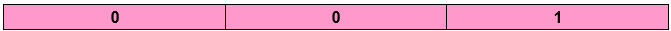#2 Operator: Right shift

Explanation: It shifts each bit in operand to right. Number of bits shifted is depended on the number following the operand. Say, ch>>4 will shift all bits in ch four places to right. Let us consider an example, variable ch has the bit pattern 111 0111 then ch>>4 will result into 000 0111.

Bit pattern for ch variable: 111 0111ch>>4

Result: 000 0111#3 Operator: Left shift

Explanation: This is similar to right shift. Instead shifting bits to right they shifted to left. Blank placeholders are filled by 0's as the right shift. It shifts each bit in operand to left. Number of bits shifted is depended on the number following the operand. Say, ch<<4 will shift all bits in ch four places to left. Let us consider an example, variable ch has the bit pattern 111 0111 then ch<<4 will result into 111 0000.

Bit pattern for ch variable: 111 0111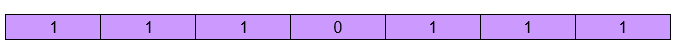ch<<4

Result: 111 0000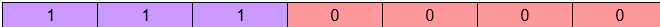#4 Operator: Bitwise AND

Explanation: This is different from logical AND. This operator operates on individual bit although the basic rule is same. Result is true only when both binary bits are of same value otherwise, false. The true bits on the result are indicated so that it becomes easy to identify Let us consider the following bit patterns then it will become clear

Variable 1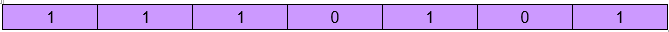Variable 2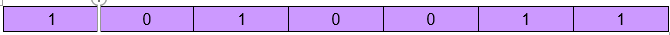Result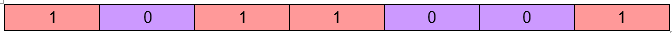#5 Operator: Bitwise OR

Explanation: This is different from logical OR. This operator operates on individual bit although the basic rule is same. Result is true when any of the binary bits is true, otherwise false. The true bits on the result are indicated so that it becomes easy to identify Let us consider the following bit patterns then it will become clear.

Variable 1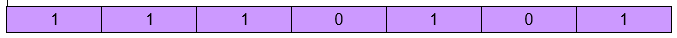Variable 2Result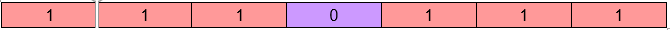#6 Operator: Bitwise XOR

Explanation: This operator returns true only when one of the binary bits is 1. This is different from OR operation. OR returns true when any one of the binary bits is 1. Following example will clear your doubt. True or binary 1 is indicated specifically so that you can easily differentiate.

Variable 1Variable 2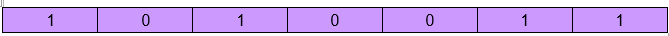Result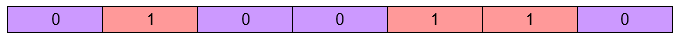## 5.9 Special operators

In this section we shall study two operators and they are as follows:

• Comma operator
• sizeof operator

### 5.9.1Comma operator

Comma operator accepts two or more operands hence is used to combine two or more statements into one statement. This is also left associative. Let us consider an example so that we can have a simple idea about the operation of this operator.

Example: int a=5;

a=temp;

int temp=c;

Explanation: All of these statements can be combined into one as shown below:

int a=temp, c, 5;

### 5.9.2 sizeof operator

The sizeof operator returns the number of bytes occupied by operand in the memory.

Format: sizeof (operand) ;

Example: sizeof (char)

Explanation: Result will be one byte as char data type occupies one byte in memory.

That's all folks we have covered almost all the operators used in C language. Now we will see some programs so that you can try them at home.

Program #1: /* Program to illustrate the arithmetic operators */

```# include <stdio.h>
# include <math.h>
# include <conio.h>

void main ()
{
int x=5, y=10;
int add, subtract, divide, multiply, remainder;
subtract=x-y;
divide=x/y;
multiply=x*y;
remainder=x%y;

printf ("Multiplication =%d\n", multiply);
printf ("Subtraction =%d\n", subtract);
printf ("Division =%d\n", divide);
printf ("Remainder=%d\n", remainder);
}
```

Output:

Subtraction=-5

Division=0

Multiplication=50

Remainder=5

Following snapshots shows the input and output of the above program: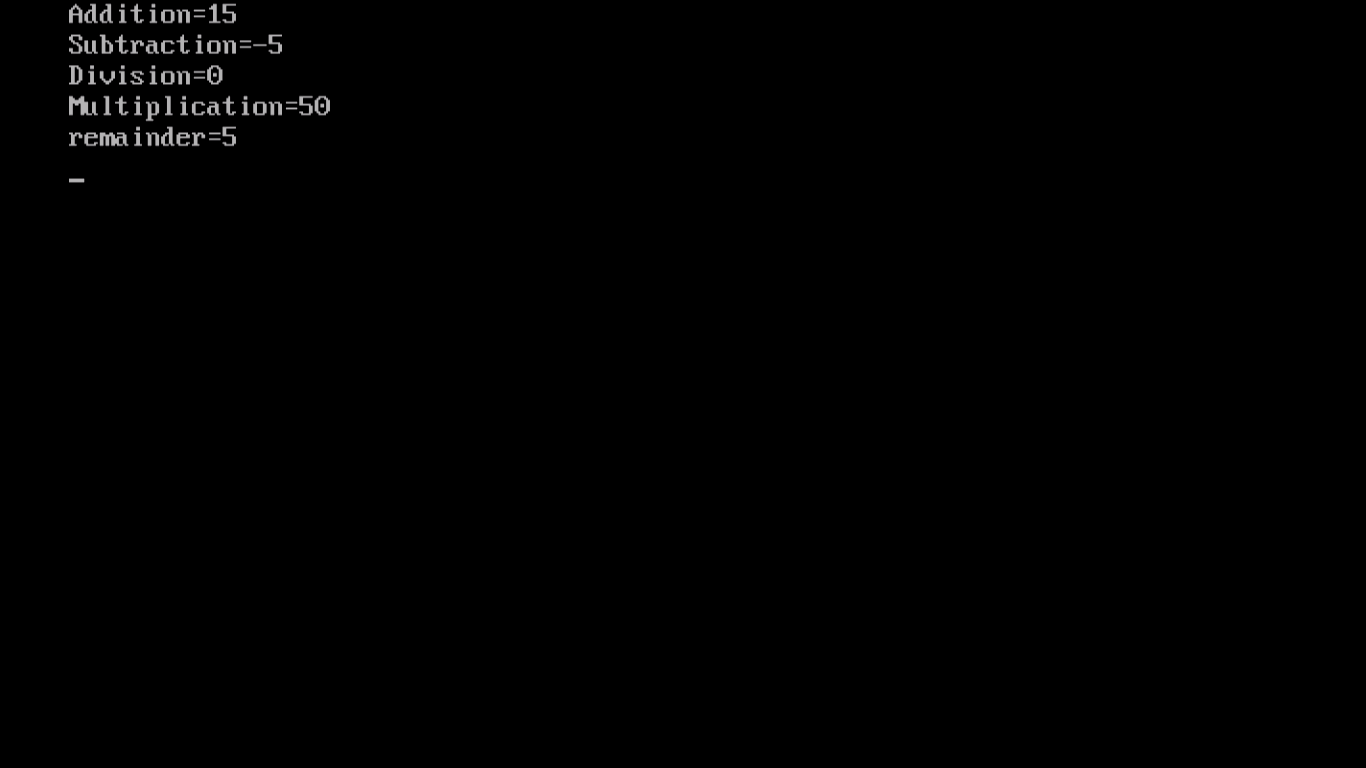Program #2: /* Program to illustrate decrement and increment operators*/

```#include<stdio.h>
#include<math.h>
#include<conio.h>

void main ()
{
int a=5, b=6;
int sum;
sum = a + b;
printf("sum = %d\n", sum);
printf("a = %d\n", ++a);
printf("b = %d\n", b++);
printf("sum = %d\n", sum);
printf("a = %d\n", --a);
printf("sum = %d\n", sum);
printf("b = %d\n", b--);
getch();
}
```

Output

sum =11

a =6

b =6

sum =11

a =5

sum =11

b =7

Following snapshots are for your reference: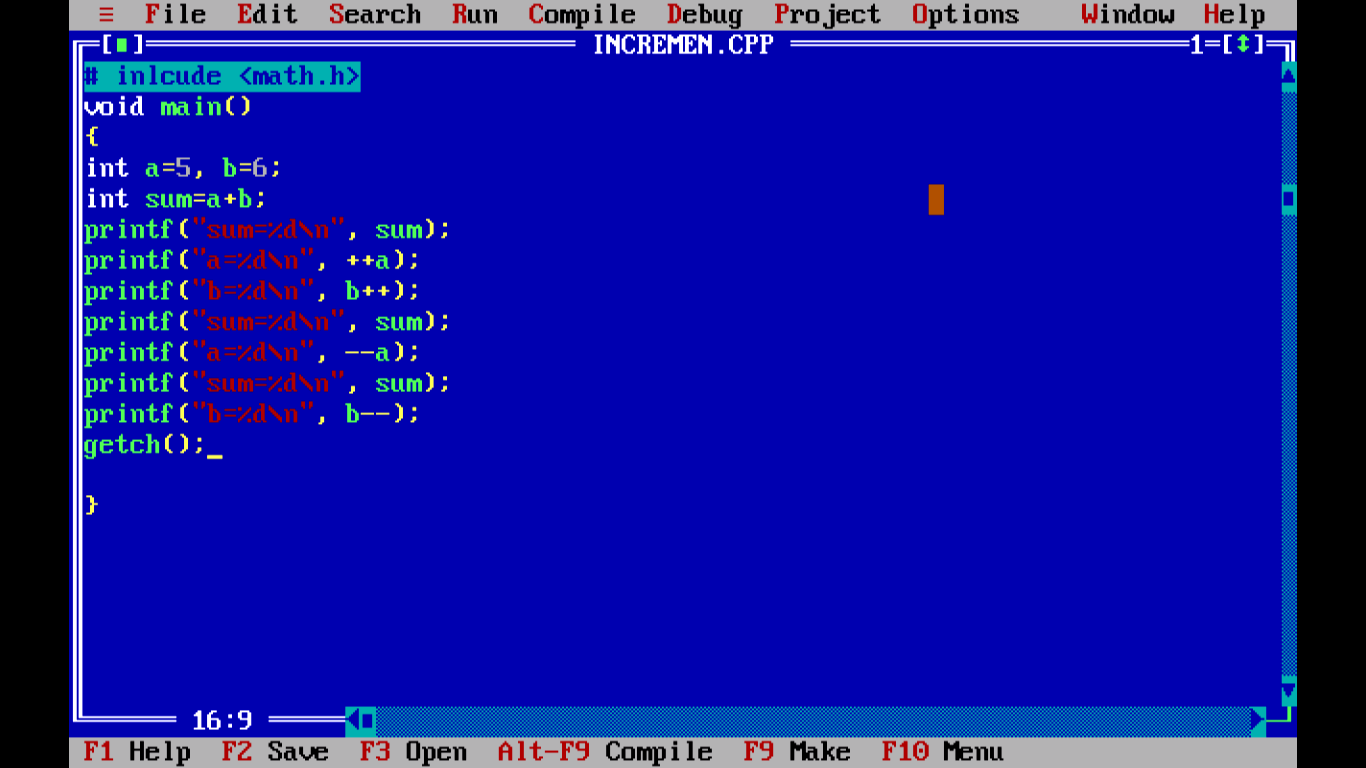Figure Program in editor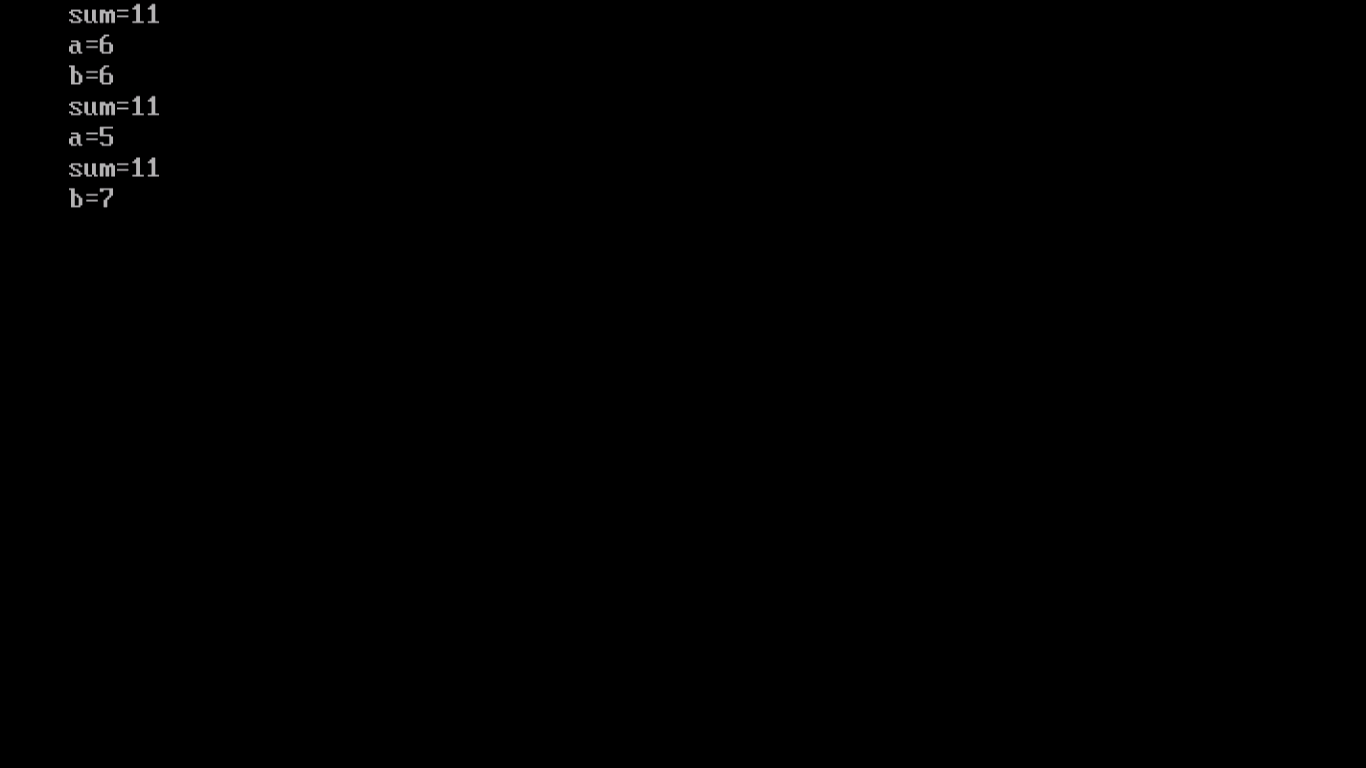Figure Output of program

Program #3: /* Program to illustrate relational operators, logical operators and conditional operators*/

```#include<stdio.h>
#include<math.h>
#include<conio.h>

void main()
{
int x=5, y=7;

/*Relational operator*/
if (x==y){
printf("They are equal\n");
}
else
{
printf("They are not equal\n");
}

/* Logical operator*/
int c=x&&y;
printf("c=%d\n", c);

/*Conditional operator*/
int result=x>y?x:y;
printf("result=%d\n", result);
getch();
} ```

Output

They are not equal

c=1

result=7

Following snapshot shows the output in the compilerFigure output of program

We are concluding this chapter. In the next chapter, we will introduce decision control structure used in C program. Thank you!!